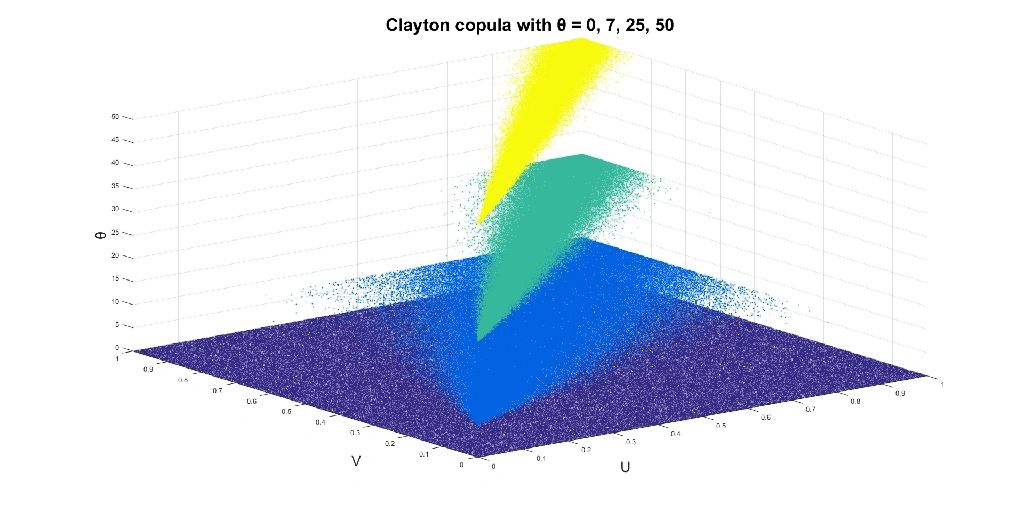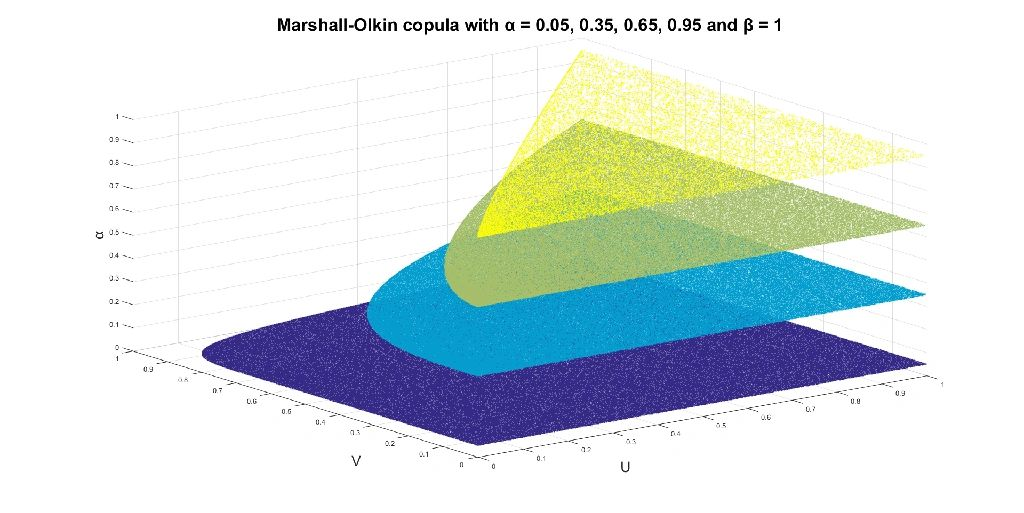Statistical & Financial Consulting by Stanford PhD
COPULA

1. Introduction

Copula is the joint distribution function of a collection of random variables U1, ..., Ud such that each of then is uniformly distributed on [0,1]. Even though the marginal distributions are fixed, the copula can take a variety of forms because variables U1, ..., Ud may have strong codependence or no codependence at all, they may be connected in a continuous or discrete fashion, they may exhibit stronger codependence in the tails or stronger dependence in the middle of the distribution. By itself copulas would represent little interest if not for the following result.

Theorem (Sklar): Let X1, ..., Xd be random variables with any marginal distribution functions F1(x), ..., Fd(x). Then H(x1,...,xd) is the joint distribution function of X1, ..., Xd if and only if there exists a copula C(u1,...,ud) such that

If X1, ..., Xd have continuous distributions, copula C(u1,...,ud) is unique.

This result makes copulas popular in modeling correlated phenomena because they provide a nice decoupling option. The modeling process can be decoupled into two completely separate stages: 1) building a separate model for each phenomenon, simple or complex but unrelated to other modeling efforts, and 2) choosing a copula to govern the joint behavior, ensuring strong correlation in some parts of the system and low correlation in others. For example, an actuary is analyzing a portfolio of insurance policies. Each of them has different duration, contractual provisions, type of client and risk factors. Some policies may call for relatively simple modeling, as primitive as extrapolating and adjusting historical life tables. Some policies may require modeling based on several correlated stochastic processes, calibrated to data in different markets or even fields. Simple or complex, actuarial methods have gone a long way to suggest what to do with each policy. So on the policy-by-policy basis the problem is solved. This does not satisfy the actuary, however, because he/she knows that all of those insurance payments are sensitive to joint risk factors, like hurricanes, recession or war. Not all of the payment will necessarily be triggered by these macro "upsets" but their likelihood or the severity of stipulated payments may certainly increase. The possibility of making many payments within a relatively short period of time is certainly a concern for the insurance company as the whole. The higher the probability of such adverse scenario, the lower the risk limits should be and/or larger amounts of cash should be set aside as the reserve. To calculate the exact amounts, the actuary must model correlation among the separate insurance plans. He/she does that by combining the previously made inferences about individual plans into one big model using a suitable copula.

Or the context of copula usage may be somewhat less polished. Rarely does the researcher sit at the table at the beginning of the modeling process and plans all the stages ahead, in a careful and consistent manner. Research groups in the industry have notoriously little human resources to address all the aspects of the problem most accurately even if exploiting the know-how in the public domain (let alone any proprietary efforts). If some parts of the system have already been modeled by other desks or outside vendors, it may be a blessing to just use their infrastructure, tweaking it a bit perhaps. The infrastructure can be combined with original research of the remaining parts of the system. All in one copula.

In other cases, there are very good reasons for using the output of other professionals, because they may be true experts in certain segments which are only components in your product. Or they may have access to the information that you do not have. So it is best to absorb their modeling insights at the fullest and combine with whatever you have managed. Say, an investment bank needs to price an interest-rates-commodity hybrid. This a derivative which is sensitive to movements in the interest rates term structure as well as the future price of a certain commodity. Separately, the IRP desk has already modeled the interest rate term structure quite well. And their traders tune the parameters daily. Separately, the commodities desk has already modeled the price dynamics of the commodity. And their traders tune the parameters several times a day. All that know-how can and must be combined, in one hybrid model. A copula is not the only way to do it (and often not the best one) but in many situations it is a competitive method, especially when considering speed and numerical stability of the implementation.

For all the reasons mentioned above, copulas have gained prominence in actuarial science, financial derivatives pricing, engineering and bioinformatics... Since codependence of extreme events is important, special measures quantifying this codependence have been introduced. For coordinates ui and uj of copula C(u1,...,ud), the lower tail dependence and upper tail dependence are defined as

respectively. If random variables X1, ..., Xd are described by copula C(u1,...,ud), then quantifies the tendency of Xi and Xj to take extremely low values together. Likewise, quantifies the tendency of Xi and Xj to take extremely high values together. Note that and

2. Mathematical Results

Copulas are directly related to two prominent measures of nonlinear association (nonlinear dependence) between two variables. If random variables X and Y have continuous marginal distributions and copula C(u,v), then their Kendall's tau and Spearman's rho are given by

respectively.

When working with copulas, simplicity and numerical tractability may prove to be important. For that reason the so-called Archimedean copulas have received much attention. An Archimedean copula has the form:

where function is known as the generator and has the following properties: , , and is decreasing and convex. Archimedean copulas have an important "divisibility" property. If d random variables are described by an Archimedean copula, then any k-element subset of them has the k-variate copula from the same Archimedean family (same generator ).

There are many well-researched families of copulas, with different properties and character. Below I am listing the most popular ones. As you will notice, they are not ideal, quite detached from reality, in fact. All of them have symmetric nature in the sense that each two random variables have the same shape of the joint distribution, albeit governed by different parameters (generally speaking). Nonetheless, the listed copulas are utilized frequently in statistical modeling because they are relatively transparent and computationally tractable. Whenever researchers can afford to look into more complex structures, they play with copulas where the conditional distribution of X1 given X2 may be very different from the conditional distribution of X2 given X1 and the two distributions may not even fall into any of the standard distributional families. For that reason, "taxonomists" single out two overlapping classes of copulas: asymmetric copulas, where each two bivariate relationships may have different shapes and/or parameter values, and empirical copulas, where the joint distribution is estimated from the data and does not belong to any standard, smooth and well-behaved distributional family. The cases below are "goody goody" though.

1] Gaussian copula (normal copula):

where is the inverse of the standard normal distribution function and is the joint distribution function of d-dimensional normal distribution with correlation matrix As you may have guessed, this is the copula generated by multivariate normal distribution with correlation matrix The most utilized case has the same correlation ρ between each two variables.

2] Student copula (t-copula):

where is the inverse distribution function of Student distribution with degrees of freedom and is the joint distribution function of d-dimensional Student distribution with correlation matrix and degrees of freedom. Not surprisingly, this is the copula generated by multivariate Student distribution with correlation matrix and degrees of freedom. The most utilized case has the same correlation ρ between each two variables.

3] Gumbel-Hougaard copula:

This is an Archimedean copula with generator

4] Clayton copula:

This is an Archimedean copula with generator Bivariate Clayton copula is visualized below for a few selected cases. To see more plots, please click the links inside the table at the bottom of this page.5] Frank copula:

This is an Archimedean copula with generator

6] Marshall-Olkin copula:

Let be a mapping from all non-empty subsets of into non-negative numbers. This mapping is one big, multi-dimensional parameter characterizing the Marshall-Olkin copula. Consider the following algorithm.
• Simulate independent random variables from U([0,1]). This gives us one random variable per each non-empty subset of

• Set
• Set
• Set
The d-variate Marshall-Olkin copula is the joint distribution function of The implied bivariate copula for any two variables can be written in a simpler form:

where

The Marshall-Olkin copula arises naturally in the study of systems reliability. Consider a d-component system where each non-empty subset of components receives a fatal shock according to an independent Poisson process with intensity Then the survival times of components are governed by a Marshall-Olkin copula... Bivariate Marshall-Olkin copula is visualized below for one special and very interesting case. To see more plots, please click the links inside the table at the bottom of this page.All the aforementioned copula families have the property that any two variables are characterized by a bivariate copula from the same copula family. Therefore, without any ambiguity we can summarize the relevant bivariate association measures in the table below. To remind the reader, the most important bivariate association measures are Kendall's tau, Spearman's rho, lower tail dependence and upper tail dependence.

 COPULA PLOTS Gaussian 0 0 2D 3D Student 2D 2D 3D Gumbel-Hougaard 0 2D 3D Clayton 0 2D 3D Frank 0 0 2D 3D Marshall-Olkin 0 2D 2D 3D
Bivariate relationships in copula families: τK = Kendall's tau, ρS = Spearman's rho, λ- = lower tail dependence, λ+ = upper tail dependence,
is the Debye function, is the distribution function of Student distribution with degrees of freedom. Whenever the cell is blank, no closed form formula is known, besides the general expressions already given.

3. Statistical Software

R: copula, VineCopula, CDVine - packages

Matlab: copulacdf, copulaparam, copulapdf, copularnd, copulastat - commands

SAS: proc copula, proc model - procedures

COPULA REFERENCES

Nelsen, R. B. (2006). An Introduction to Copulas (2nd ed). New York: Springer.

Salvadori, G., De Michele, C., Kottegoda, N. T., & Rosso, R. (2007). Extremes in Nature: An Approach Using Copulas. Springer.

Embrechts, P., Lindskog, F., & McNeil, E. J. (2001). Modelling Dependence With Copulas and Applications to Risk Management. Working paper.

Jaworski, P., Durante, F., Härdle, W.K., & Rychlik, T. (2010). Copula Theory and Its Applications. Proceedings of the Workshop Held in Warsaw, 25-26 September 2009.

Cherubini, U., Gobbi, F., Mulinacci, S., & Romagnoli, S. (2011). Dynamic Copula Methods in Finance. Wiley.

Joe, H. (2014). Dependence Modeling with Copulas. Chapman & Hall / CRC. - Not an intuitive exposition of the concepts but rather an extensive dictionary of formulas and equations.

COPULA RESOURCES

BACK TO THE STATISTICAL ANALYSES DIRECTORY